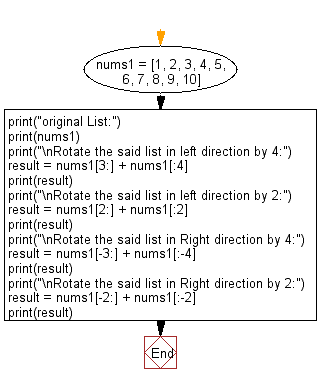﻿ Python: Rotate a given list by specified number of items to the right or left direction - w3resource

# Python: Rotate a given list by specified number of items to the right or left direction

## Python List: Exercise - 109 with Solution

Write a Python program to rotate a given list by a specified number of items in the right or left direction.

Sample Solution:

Python Code:

``````nums1 = [1, 2, 3, 4, 5, 6, 7, 8, 9, 10]
print("original List:")
print(nums1)
print("\nRotate the said list in left direction by 4:")
result = nums1[3:] + nums1[:4]
print(result)
print("\nRotate the said list in left direction by 2:")
result = nums1[2:] + nums1[:2]
print(result)
print("\nRotate the said list in Right direction by 4:")
result = nums1[-3:] + nums1[:-4]
print(result)
print("\nRotate the said list in Right direction by 2:")
result = nums1[-2:] + nums1[:-2]
print(result)
```
```

Sample Output:

```original List:
[1, 2, 3, 4, 5, 6, 7, 8, 9, 10]

Rotate the said list in left direction by 4:
[4, 5, 6, 7, 8, 9, 10, 1, 2, 3, 4]

Rotate the said list in left direction by 2:
[3, 4, 5, 6, 7, 8, 9, 10, 1, 2]

Rotate the said list in Right direction by 4:
[8, 9, 10, 1, 2, 3, 4, 5, 6]

Rotate the said list in Right direction by 2:
[9, 10, 1, 2, 3, 4, 5, 6, 7, 8]
```

Flowchart:## Visualize Python code execution:

The following tool visualize what the computer is doing step-by-step as it executes the said program:

Python Code Editor:

Have another way to solve this solution? Contribute your code (and comments) through Disqus.

What is the difficulty level of this exercise?

Test your Programming skills with w3resource's quiz.

﻿

## Python: Tips of the Day

Chunks a list into smaller lists of a specified size:

Example:

```from math import ceil

def tips_chunk(lst, size):
return list(
map(lambda x: lst[x * size:x * size + size],
list(range(0, ceil(len(lst) / size)))))

print(tips_chunk([1, 2, 3, 4, 5, 6], 3))
```

Output:

```[[1, 2, 3], [4, 5, 6]]
```